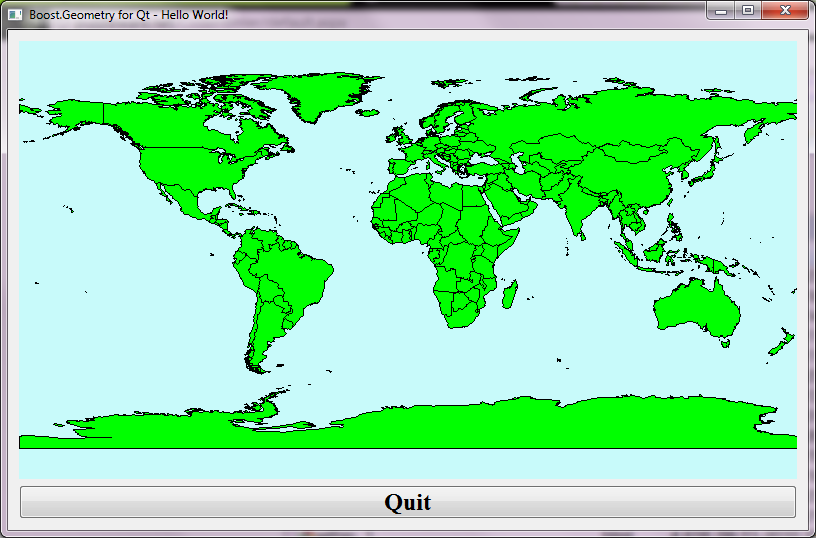# Qt World Mapper with Boost.Geometry

Last year I created a simple world mapper using WxWidgets. For various reasons I decided to make the Qt equivalent as well.

Both Qt and WxWidgets are well-known cross-platform windows frameworks. Qt is used in Quantum GIS.

The World Mapper I created with WxWidgets did read world countries from a WKT (well-known text) file, and displayed them. Moving the mouse did highlight the country. For Qt it is similar (the highlighting is not yet been done).

The nice thing is that, by setting Boost.Geometry in this context, its force is shown:

Look below to lines marked with // Adapt. The QPointF and QPolygonF are just registered. From now on they act as normal Boost.Geometry geometries. So they can be used in area calculation, distance, transform, etc. We only use transform in this example.

So also look at lines commented with // This is the essention. Here a QPolygonF is declared, a ring (a normal Boost.Geometry part of a polygon, part of a multi-polygon) is transformed using the transformer to a QPolygonF. And the last one is of course displayed without any problem.

Delivering this application:## Complete C++ Code

`#include <fstream> #include <QtGui> #include <QWidget> #include <QObject> #include <QPainter> #include <boost/foreach.hpp> #include <boost/geometry/geometry.hpp> #include <boost/geometry/geometries/register/point.hpp>#include <boost/geometry/geometries/register/ring.hpp>#include <boost/geometry/multi/multi.hpp> #include <boost/geometry/extensions/algorithms/selected.hpp>#include <boost/geometry/extensions/gis/io/wkt/wkt.hpp> // Adapt a QPointF such that it can be handled by Boost.GeometryBOOST_GEOMETRY_REGISTER_POINT_2D_GET_SET(QPointF, double, cs::cartesian, x, y, setX, setY)// Adapt a QPolygonF as well. // A QPolygonF has no holes (interiors) so it is similar to a Boost.Geometry ringBOOST_GEOMETRY_REGISTER_RING(QPolygonF) typedef boost::geometry::model::d2::point_xy<double> point_2d; typedef boost::geometry::model::multi_polygon     <         boost::geometry::model::polygon<point_2d>     > country_type; class WorldMapper : public QWidget{ public:     WorldMapper(std::vector<country_type> const& countries, boost::geometry::model::box<point_2d> const& box)        : m_countries(countries)         , m_box(box)     {         setPalette(QPalette(QColor(200, 250, 250)));        setAutoFillBackground(true);     } protected:     void paintEvent(QPaintEvent*)     {         map_transformer_type transformer(m_box, this->width(), this->height());         QPainter painter(this);         painter.setBrush(Qt::green);         BOOST_FOREACH(country_type const& country, m_countries)        {           typedef boost::range_value<country_type>::type polygon_type;          BOOST_FOREACH(polygon_type const& polygon, country)          {            typedef boost::geometry::ring_type<polygon_type>::type ring_type;           ring_type const& ring = boost::geometry::exterior_ring(polygon);           // This is the essention:            // Directly transform from a multi_polygon (ring-type) to a QPolygonF            QPolygonF qring;            boost::geometry::transform(ring, qring, transformer);            painter.drawPolygon(qring);           }        }     } private:     typedef boost::geometry::strategy::transform::map_transformer        <           point_2d, QPointF,           true, true         > map_transformer_type;      std::vector<country_type> const& m_countries;     boost::geometry::model::box<point_2d> const& m_box;}; class MapperWidget : public QWidget{ public:      MapperWidget(std::vector<country_type> const& countries, boost::geometry::model::box<point_2d> const& box, QWidget *parent = 0)         : QWidget(parent)      {          WorldMapper* mapper = new WorldMapper(countries, box);         QPushButton *quit = new QPushButton(tr("Quit"));          quit->setFont(QFont("Times", 18, QFont::Bold));          connect(quit, SIGNAL(clicked()), qApp, SLOT(quit()));          QVBoxLayout *layout = new QVBoxLayout;          layout->addWidget(mapper);          layout->addWidget(quit);          setLayout(layout);      } }; template <typename Geometry, typename Box>inline void read_wkt(std::string const& filename, std::vector<Geometry>& geometries, Box& box){     std::ifstream cpp_file(filename.c_str());     if (cpp_file.is_open())     {         while (! cpp_file.eof() )         {           std::string line;           std::getline(cpp_file, line);           if (! line.empty())           {               Geometry geometry;               boost::geometry::read_wkt(line, geometry);               geometries.push_back(geometry);               boost::geometry::combine(box, boost::geometry::make_envelope<Box>(geometry));           }         }     } } int main(int argc, char *argv[]){     std::vector<country_type> countries;     boost::geometry::model::box<point_2d> box;     boost::geometry::assign_inverse(box);     read_wkt("../data/world.wkt", countries, box);     QApplication app(argc, argv);     MapperWidget widget(countries, box);     widget.setWindowTitle("Boost.Geometry for Qt - Hello World!");     widget.setGeometry(50, 50, 800, 500);    widget.show();     return app.exec(); }`

## Conclusions

With less than 160 lines of code, Qt and Boost.Geometry we showed how it can quite conveniently combine together.
The source is shown above and in SVN it can be found here.

Actually this application (and its WxWidgets counterpart) was for me the reason to start the shapefile research, which final conclusions still have to be drawn.